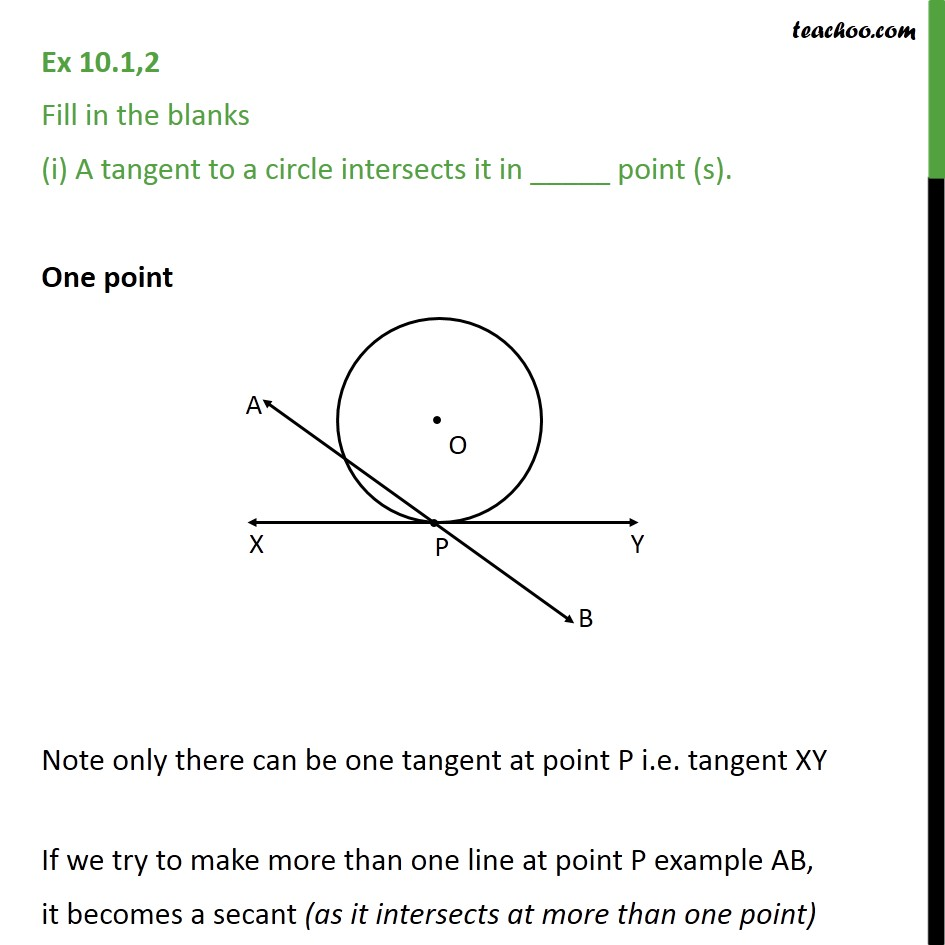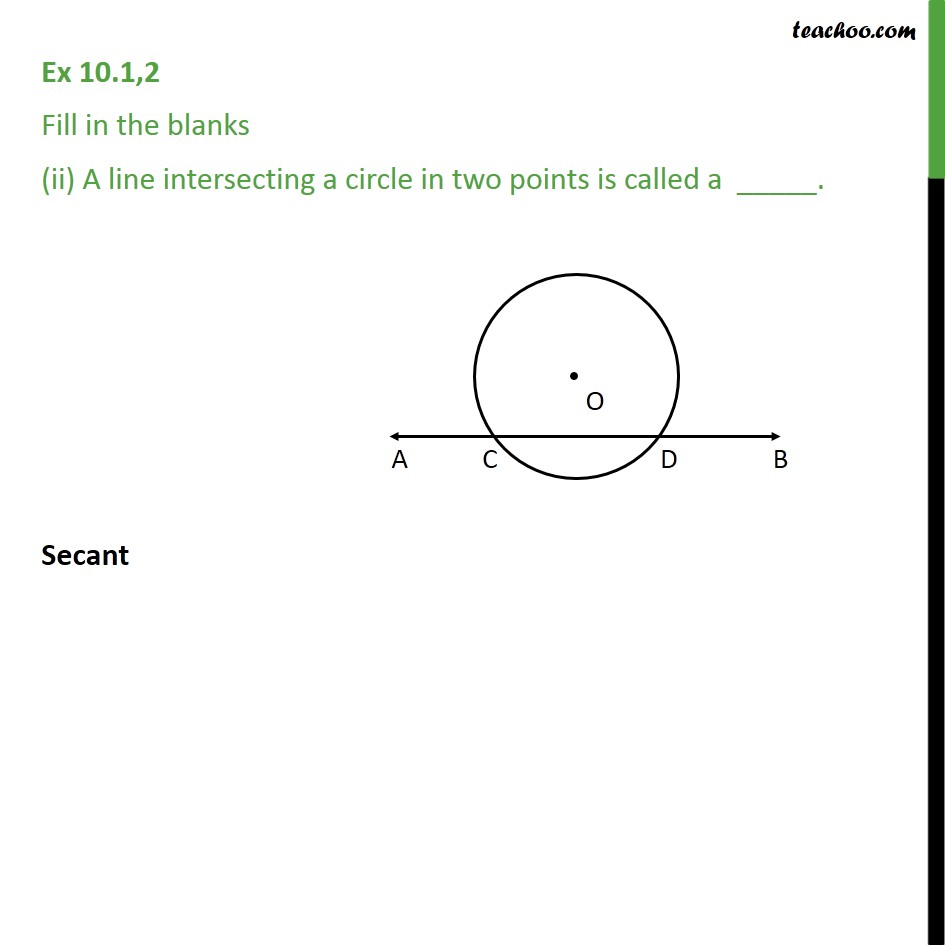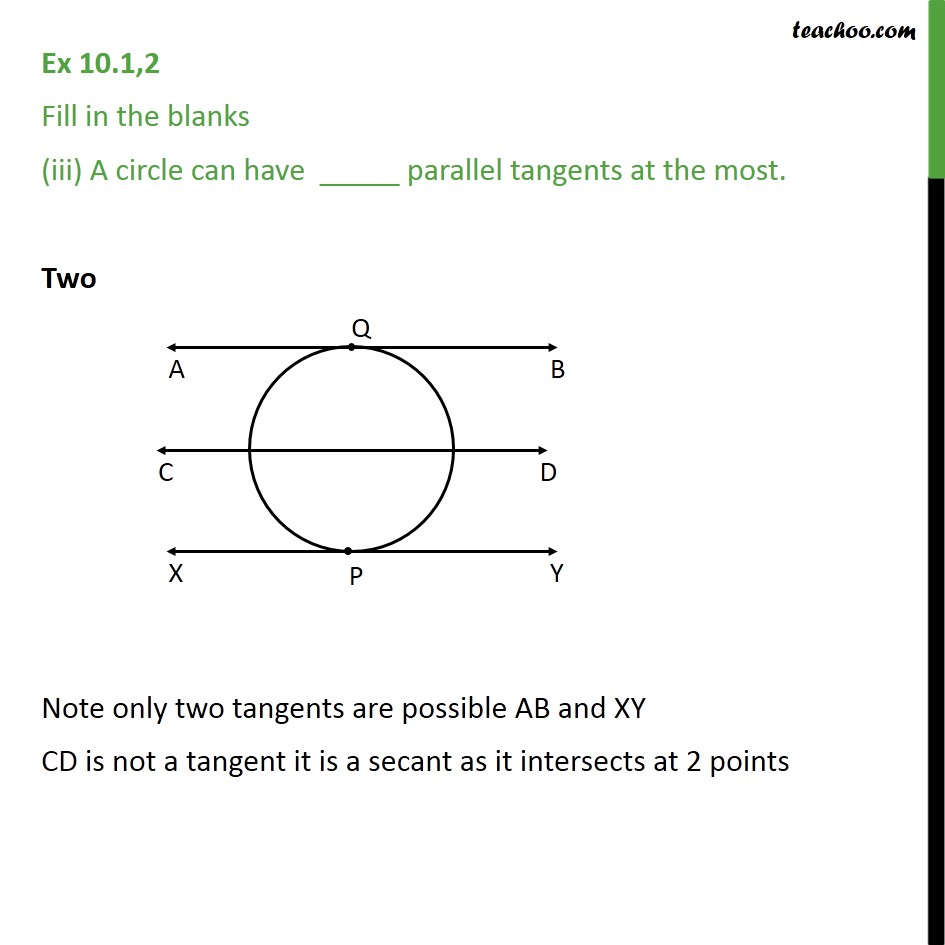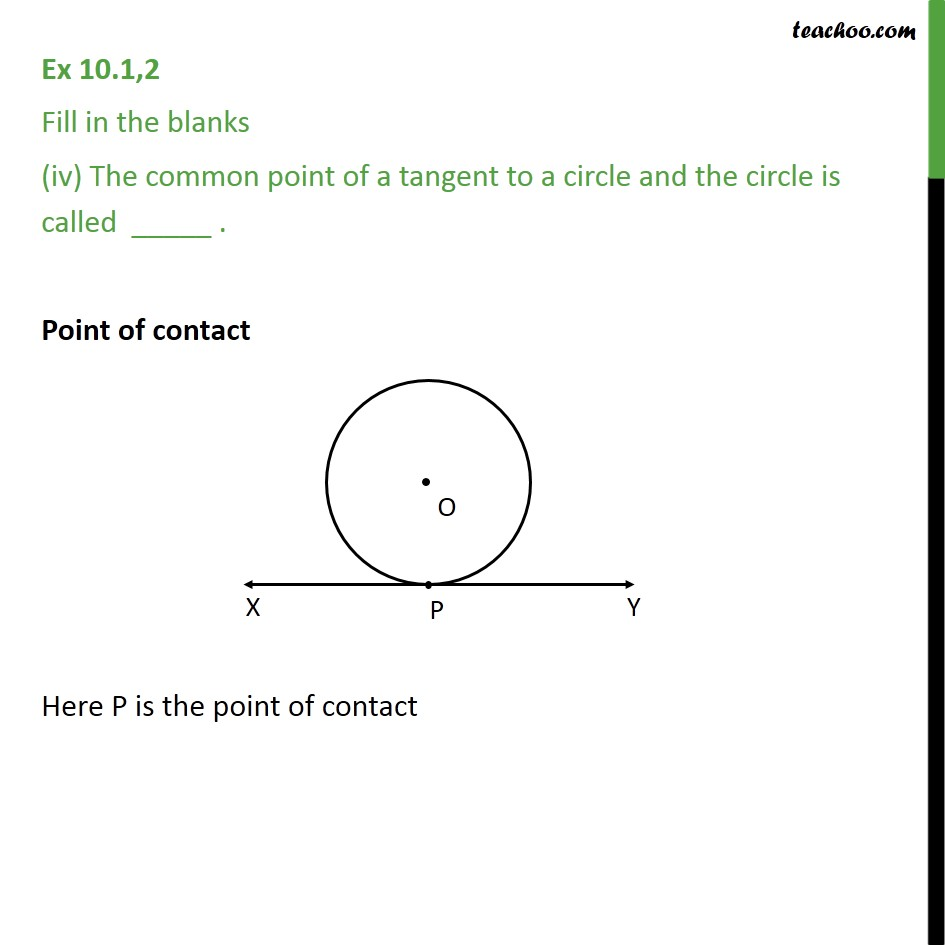Ex 10.1

Chapter 10 Class 10 Circles
Serial order wiseLearn in your speed, with individual attention - Teachoo Maths 1-on-1 Class

### Transcript

Ex 10.1,2 Fill in the blanks (i) A tangent to a circle intersects it in _____ point (s). One point Note only there can be one tangent at point P i.e. tangent XY If we try to make more than one line at point P example AB, it becomes a secant (as it intersects at more than one point) Ex 10.1,2 Fill in the blanks (ii) A line intersecting a circle in two points is called a _____. Secant Ex 10.1,2 Fill in the blanks (iii) A circle can have _____ parallel tangents at the most. Two Note only two tangents are possible AB and XY CD is not a tangent it is a secant as it intersects at 2 points Ex 10.1,2 Fill in the blanks (iv) The common point of a tangent to a circle and the circle is called _____ . Point of contact Here P is the point of contact